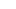# All You Need to Know About GRE Quant (Geometry)In GRE, Geometry takes up approximately 15% to 20% of the Quant section. That would be approximately 6 to 8 questions, not including the experimental questions.

Geometry is a vast topic in Math including all kinds of shapes, measurements, theorems, etc., but in GRE Quant, it is restricted to basic shapes (triangles, quadrilateral, etc.) and straight lines in geometry. They rarely test curves (parabola) in co-ordinate geometry. GRE Geometry mostly tests the visual skills and basic measurements (area, angles, perimeter, etc.)

Most of the Indian students do well in GRE Geometry because they are good in basic shapes and formulae.

#### What is tested in Geometry?

What is tested in Geometry can be split into three broad buckets:

• Lines and Angles

• Circles and 3D

• Co-ordinate geometry

### I) Lines and Angles

Lines and Angles test your skills at:

• Parallel and perpendicular lines

• Angles of two or more parallel lines

• Properties of parallel and perpendicular lines

#### Sample questionIn the figure above, what is the value of r + s?

1. 112

2. 118

3. 122

4. 128

5. 142

#### Explanation

The sum of the interior angles of a triangle is 180 degrees

So, in triangle ACD, angle CAD = 52 degrees

90 = 52+/DAB

So /DAB = 38

Since r = 90

So r+s = 128

### II) Polygons (Convex)

A GRE student should be aware of all the basic rules of triangles and quadrilaterals (basic polygons).

• Sum of the interior and exterior angles of a polygon

• Area and perimeter of a triangle.

• Different types of triangles (with both sides and angle wise)

• Special triangles 30 – 60 – 90 and isosceles right angle triangle.

• Similar triangles.

• Third Side Rule of a triangle

• Area and perimeter of a Quadrilateral

• Angles and Diagonals properties in special quadrilaterals

#### Explanation

Remember the third side rule of a triangle.

The difference of the other two sides < Third Side of any triangle < Sum of other two sides

So here, the third side has to be between.

5 < Third Side < 11

Only statement II is true.

### III) Circles and 3D

Circles in the GRE test your skills at:

• Finding the area and circumference of the circle

• Finding the arc length and area covered by an arc (sector area)

• Central angle theorem

• Tangents

• Chords

• Inscribing polygons (circle inside a square or rectangle, or vice versa)

GRE Three dimensional geometry, tests only the basic shapes like rectangular solids, cylinder and sphere.

3D in the GRE tests your skills at:

• Volume and surface area of the cube and cuboids

• Volume and surface area of the cylinder and sphere

• Diagonal and center of rectangular solids, cylinder and sphere

#### Sample Question#### Explanation

From the diagram:

OPQ is an isosceles triangle because OP = OQ as they are the radius of the given circle.

So, /OPQ = /PQO = y

We know that the sum of the interior angles of a triangle is 180.

Given,

2y + x = 180

0 < x < 40

2y > 140

y > 70

Also, 2y < 180

So y < 90

Therefore, 70<y<90

IV) Co – ordinate Geometry

Co – ordinate Geometry in GRE tests your skills at:

• Equation of a line

• Distance between two points

• Slope of a line (also slope of parallel and perpendicular lines)

• Finding x and y intercepts

• Special lines passing through the origin(y = x and y = -x)

• Reflection of a point across x and y axes

• Midpoint of a line

• Intersection of two lines

#### Explanation

As the vertices points given are of a square, all sides should be equal.

Let’s plot these points in the co-ordinate plane:From this diagram, we can see that the diagonals intersect each other in the fourth quadrant at (1/2, -1/2).